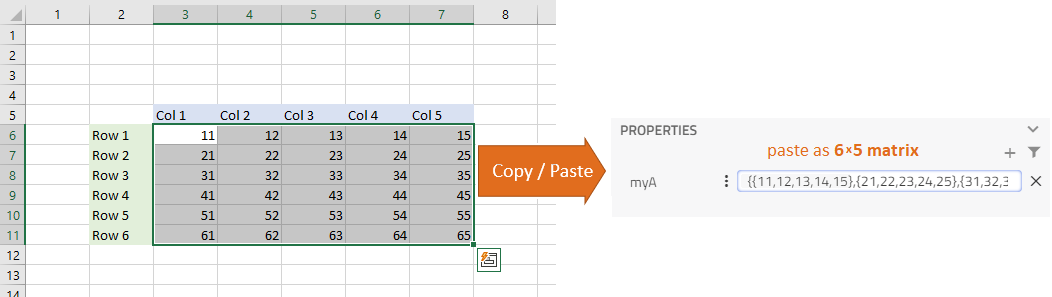# Import Data from Excel in Parameters🔗

Impact supports the direct insertion of data from Excel when in modeling mode.

This is a simple way to parameterize vectors or matrices by given data via the input field of parameters and variables in the Properties panel.

1. Open a spreadsheet in Excel (CSV or XLSX), which contains your numerical data
2. Select the area that represents the matrix (2d table) or vector (row)
3. Copy
4. Paste it into the empty input field

Impact will automatically convert the clipboard data into Modelica-compliant code. This functionality supports: vectors `[:]` (rows), 2D matrices `[:,:]` (tables) or scalar values (single cell content). Thereby the `size` of each dimension is not limited, but note, pasting very large amounts of data affects performance.

Copy Excel data, paste in the model parameterNote

• The copy of a column of data will be transformed into a matrix of dimension `[:,1]`
• The copy of a row of data will be transformed into a vector of dimension `[:]`
• If you only copy the content of a single cell, then the content can be pasted anywhere (even in the code editor)

The current version of Impact has the following restrictions:

• Make sure that the source data contains a *dot as decimal separator and *commas as thousands separator
• Make sure that all cell data in the source have the same data type (`Real` or `Integer` (a subset of Real)). Do not mix, as it is not allowed in Modelica
• All cells within a selection must contain data (empty cells would break the Modelica array)
• Make sure not to copy table headers or similar items
• Pasting `Strings`, `Booleans`, or mathematical expressions like `x+4` are not supported